## 将方程插入实时编辑器中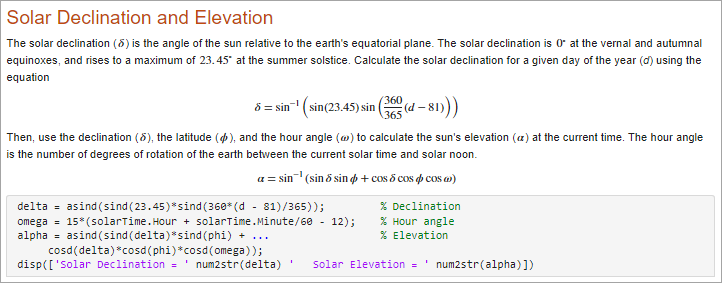• 以交互方式插入方程 - 您可以通过从符号和结构体的图形显示中进行选择，从而以交互方式构建方程。

• 插入 LaTeX 方程 - 您可以输入 LaTeX 命令，实时编辑器就会插入对应的方程。

### 以交互方式插入方程

1. 转到插入选项卡，然后点击此时将会出现一个空白方程。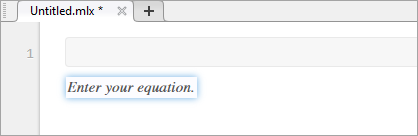2. 方程选项卡显示的选项中选择符号、结构体和矩阵以构建方程。点击各部分右侧的下拉箭头以查看其他选项。

添加或编辑矩阵时，将会显示一个上下文菜单，您可以使用该菜单来删除和插入行与列。您也可以使用上下文菜单来更改或删除矩阵分隔符。

3. 使用文本部分中提供的选项来设置方程格式。格式设置仅适用于方程中的文本。无法设置数值和符号的格式。除非将光标放在可设置格式的文本中，否则格式设置选项将处于禁用状态。

#### 用于方程编辑的键盘快捷方式

• 要插入符号、结构体和矩阵，请键入一个反斜杠，后跟符号的名称。例如，键入 \pi 以在方程中插入 π 符号。要发现符号或结构体的名称，请悬停在方程选项卡中的对应按钮的上方。您也可以在方程编辑器中键入反斜杠，以显示所有支持名称的自动填充菜单。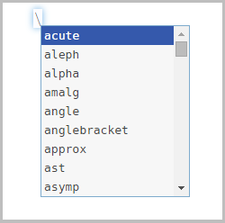注意

尽管 \name 语法与 LaTeX 命令语法非常相似，但在以交互方式插入方程时不支持输入完整的 LaTeX 表达式。

• 要插入下标、上标和分数，请使用符号 ‘_’、‘^’ 或 ‘/’。例如：

• 键入 x_2 可将 ${x}_{2}$ 插入到方程中。

• 键入 x^2 可将 ${x}^{2}$ 插入到方程中。

• 键入 x/2 可将 $\frac{x}{2}$ 插入到方程中。

• 要将新列插入矩阵，请在矩阵行中的最后一个元胞的末尾键入 ‘,’。要插入新行，请在矩阵列中的最后一个元胞的末尾键入分号 ‘;’。

• 要插入下表中列出的常用符号，请键入其他符号的组合。

键盘输入符号键盘输入符号键盘输入符号
| |=>!=
|=<-->!<
|–<->!>
–|<=!<=
->>=!>=
<-<>
<--~=

### 插入 LaTeX 方程

1. 转至插入选项卡，点击并选择 LaTeX 方程

2. 在显示的对话框中输入 LaTeX 表达式。例如，您可以输入 \sin(x) = \sum_{n=0}^{\infty}{\frac{(-1)^n x^{2n+1}}{(2n+1)!}}

预览窗格显示实时脚本中显示的方程预览。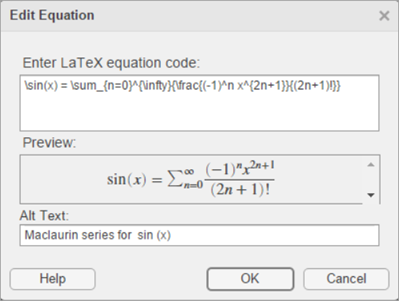3. 要在将实时脚本导出到 HTML 时包含 LaTeX 方程的说明，请将文本添加到替换文本字段中。例如，您可以输入文本 Maclaurin series for sin(x)

该说明为方程指定替换文本，并作为 alt 属性保存在 HTML 文档中。例如，在用户使用屏幕阅读器时，它可以提供有关方程的附加信息。

4. 将方程插入实时脚本中。

LaTeX 表达式描述了各种方程。下表显示了多个 LaTeX 表达式示例及其在插入实时脚本中以后的外观。

LaTeX 表达式实时脚本中的方程
a^2 + b^2 = c^2${a}^{2}+{b}^{2}={c}^{2}$
\int_{0}^{2} x^2\sin(x) dx${\int }_{0}^{2}{x}^{2}\mathrm{sin}\left(x\right)dx$
\sin(x) = \sum_{n=0}^{\infty}{\frac{(-1)^n x^{2n+1}}{(2n+1)!}}$\mathrm{sin}\left(x\right)=\sum _{n=0}^{\infty }\frac{{\left(-1\right)}^{n}{x}^{2n+1}}{\left(2n+1\right)!}$
{a,b,c} \neq \{a,b,c\}$a,b,c\ne \left\{a,b,c\right\}$
x^{2} \geq 0\qquad \text{for all}x\in\mathbf{R}
\matrix{a & b \cr c & d}$\begin{array}{cc}a& b\\ c& d\end{array}$

#### 支持的 LaTeX 命令

MATLAB 支持大多数标准 LaTeX 数学模式命令。这些表显示了支持的 LaTeX 命令列表。

øoœoeåaa
ØOŒOEÅAA
łlæaeßss
ŁLÆAE

αalphaνnuξxi
βbetaωomega ζzeta
χchiоomicronɛvarepsilon
δdeltaϕphiφvarphi
εepsilonπpi ϖvarpi
ηetaψpsiϱvarrho
vgamma ρrhoςvarsigma
ιiotaσsigma $\vartheta$vartheta
κkappaτtauאaleph
λlambda θtheta
μmuυupsilon
ΔDelta ΦPhiΘTheta
ΓGammaΠPi ϒUpsilon
ΛLambda ΨPsiΞXi
ΩOmega ΣSigma

*ast±pmwr
starmpcap
$·$cdot⨿amalgcup
circodot uplus
bulletominus sqcap
diamondoplus  sqcup
setminusoslash wedgeland
×timesotimesveelor
÷divbigoplustriangleleft
botperpbigotimestriangleright
topbigodot$△$bigtriangleup
prodbiguplus$▽$bigtriangledown
coprodbigcapbigvee
intintopbigcupbigwedge
intbarsumbigsqcup
oint

equiv <lt>gt
congleleq gegeq
neqnenot=not<not>
simprecsucc
simeqpreceqsucceq
approxllgg
asympsubsetsupset
doteqsubseteqsupseteq
proptosqsubseteqsqsupseteq
modelsmidin
bowtieparallelnotin
vdashiffniowns
dashv

leftarrowrightarrowuparrow
LeftarrowRightarrowUparrow
longleftarrowlongrightarrowdownarrow
LongleftarrowLongrightarrowDownarrow
hookleftarrowhookrightarrowupdownarrow
leftharpoondownrightharpoondownUpdownarrow
leftharpoonuprightharpoonupleftrightarrow
swarrownearrowLeftrightarrow
nwarrowsearrowlongleftrightarrow
mapstolongmapstoLongleftrightarrow

{lbrace}rbrace|vert
[lbrack]rbrackVert
langlerangle\backslash
lceilrceil
lfloorrfloor
llbracketrrbracket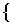bigbiglbigrbigm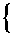biggbigglbiggrbiggm$\left\{abc\right\}$brace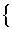BigBiglBigrBigm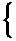BiggBigglBiggrBiggm$\left[abc\right]$brack

infty forallwp
nablaexistsangle
partialemptysettriangle
Imıilozenge
Reȷjhbar
ellıimathprime
dotsldotshellipȷjmath¬lnotneg
$\cdots$cdots:colon$\surd$surd
$\ddots$ddots$\cdot$cdotpgets
vdots$.$ldotpto
becausethereforevarpropto
°degreeclubsuitvarclubsuit
dagdaggerheartsuitvarheartsuit
ddagddaggerdiamondsuitvardiamondsuit
ldshspadesuitvarspadesuit
§mathsection

exists 命令可以与 not 命令组合使用，以创建符号的否定版本。例如，\not\exists 可创建符号 ∄。

£poundstextsterling¿textquestiondownquotedblbase
¢textcent¡textexclamdownbackprime
texteuro«flqqguillemotleft space
¥yen»frqqguillemotrightpermil
®textregistered§S\backslash
trademarktexttrademarkP¦brokenvert
©copyright

áacute$\stackrel{˙}{a}$dot$\stackrel{˜}{a}$tilde
$\overline{a}$bar$\stackrel{¨}{a}$ddot$\stackrel{\to }{a}$vec
$\stackrel{⌣}{a}$breve$\stackrel{⃛}{a}$dddotàgrave
$\stackrel{\text{ˇ}}{a}$check$\stackrel{⃜}{a}$ddddot$\stackrel{^}{a}$hat

$\mathrm{arccos}$arccos$\mathrm{det}$det$\mathrm{ln}$ln
$\mathrm{arcsin}$arcsin$\mathrm{dim}$dim$\mathrm{log}$log
$\mathrm{arctan}$arctan$\mathrm{exp}$exp$\mathrm{max}$max
$\mathrm{arg}$arg$\mathrm{gcd}$gcd$\mathrm{min}$min
$\mathrm{cos}$cos$\mathrm{hom}$hom$\mathrm{Pr}$Pr
$\mathrm{cosh}$cosh$\mathrm{ker}$ker$\mathrm{sec}$sec
$\mathrm{cot}$cot$\mathrm{lg}$lg$\mathrm{sin}$sin
$\mathrm{coth}$coth$\mathrm{lim}$lim$\mathrm{sinh}$sinh
$\mathrm{csc}$csc$\mathrm{lim}\mathrm{inf}$liminf$\mathrm{sup}$sup
$\mathrm{deg}$deg$\mathrm{lim}\mathrm{sup}$limsup$\mathrm{tan}$tan

$\frac{abc}{xyz}$frac$\stackrel{a}{b}$stackreloverset$\frac{a}{b}$over
$\sqrt{abc}$sqrt$\underset{a}{b}$underset$\left[\frac{a}{b}\right]$overwithdelims
$\text{\hspace{0.17em}}\mathrm{mod}\text{\hspace{0.17em}}a$bmod$\left(\begin{array}{c}a\\ b\end{array}\right)$binomchoose$\stackrel{←}{abc}$overleftarrow
$\text{\hspace{0.17em}}\text{\hspace{0.17em}}\text{\hspace{0.17em}}\text{ }\left(\mathrm{mod}a\right)$pmod$\left(\begin{array}{cc}a& b\\ c& d\end{array}\right)$pmatrix$\stackrel{\to }{abc}$overrightarrow
$\stackrel{^}{abc}$widehat$\begin{array}{cc}a& b\\ c& d\end{array}$matrix$\stackrel{↔}{abc}$overleftrightarrow
$\stackrel{˜}{abc}$widetilde$\begin{array}{cc}a& b\\ c& d\end{array}$begin{array}$\underset{¯}{\begin{array}{c}\overline{ab}\\ cd\end{array}}$hline
$\underset{a}{\overset{b}{\int }}$limits$\left\{\begin{array}{ll}a\hfill & b\hfill \\ c\hfill & d\hfill \end{array}$begin{cases}$\left\{\frac{a}{2}|{b}^{2}\right\}$leftmiddleright
$〈abc|$bra$|abc〉$ket$〈ab|cd〉$braket

$ab$negthinspace$abc$mathord$a\left[b$mathopen
$ab$thinspace$a\sum b$mathop$a\right]b$mathclose
$a\text{\hspace{0.17em}}b$enspace$a+b$mathbin$a\text{\hspace{0.17em}}\text{\hspace{0.17em}}|\text{ }\text{\hspace{0.17em}}\text{\hspace{0.17em}}b$mathinner
$a\text{\hspace{0.17em}}\text{\hspace{0.17em}}\text{\hspace{0.17em}}\text{ }b$quad$a=b$mathrel$a\text{\hspace{0.17em}}\text{\hspace{0.17em}}\text{\hspace{0.17em}}\text{ }b$kernmkern
$a\text{ }\text{\hspace{0.17em}}\text{\hspace{0.17em}}\text{\hspace{0.17em}}b$qquad$a,b$mathpunct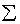displaystyle$\text{ABCDE}$texttextnormal$\text{ABCDE}$texttt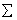textstyle$ABCDE$bftextbfmathbf$ABCDE$textsf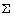scriptstyle$ABCDE$ittextitmathit$\mathcal{A}ℬ\mathcal{C}\mathcal{D}ℰ$calmathcal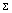scriptscriptstyle$\text{ABCDE}$rmtextrmmathrm$\mathbb{A}\mathbb{B}ℂ\mathbb{D}$mathbb
$\text{ABCDE}$hboxmbox$\mathfrak{A}\mathfrak{B}ℭ\mathfrak{D}$mathfrak